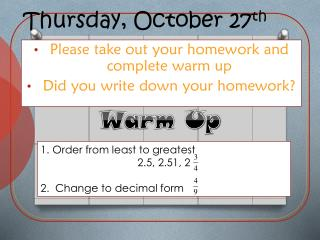DownloadDownload PresentationThursday, October 27 th

# Thursday, October 27 th

Télécharger la présentation## Thursday, October 27 th

- - - - - - - - - - - - - - - - - - - - - - - - - - - E N D - - - - - - - - - - - - - - - - - - - - - - - - - - -
##### Presentation Transcript

1. Thursday, October 27th Warm Up Please take out your homework and complete warm up Did you write down your homework? Order from least to greatest 2.5, 2.51, 2 2. Change to decimal form

2. Corny Joke of the Day Why didn’t the mummy have any friends? He was too wrapped up in himself

3. Schedule for the Week Thursday: adding and subtracting decimal integers Friday: Adding and subtracting fraction integers Tuesday: Checkpoint

4. Fraction Test Congrats to test Toppers

5. Retest? • 75 and above you will complete a packet • 74 and below you are eligible for a reteach • Tuesday 11/1 will be the reteach • Wednesday 11/2 will be the retest

6. Retest Qualifications • All Homework must from unit must be complete before you retake the test • Retest form • Retake packet must be complete before you retake the test

7. You need to tell me by Tomorrow!!!

8. Homework Answers Around the World

9. Question of the Day?!?! How do add and subtract decimals that are positive and negative?!?!

10. 66.8 + 4.0 Decimal Review Use zero as a placeholder so that both numbers have the same number of digits after their decimal points. 70.8 Add each column just as you would add integers. Line up the decimal points.

11. Negative/Positive Review Same sign add and keepDifferent sign subtractTake the sign of the farther numberThen it'll be exact! Change the minus to a plusChange the sign of nextThen all you do is add them upAs if it were a plus!

12. 4.55 + 11.30 Add. Estimate to check whether each answer is reasonable. 4.55 + 11.3 Line up the decimal points. Use zero as a placeholder. Add. 15.85 Estimate 5 + 11 = 16 15.85 is a reasonable answer.

13. 6.44 + 16.00 Add. Estimate to check whether each answer is is reasonable. 6.44 + 16 Line up the decimal points. Use zeros as placeholders. Add. 22.44 Estimate 6 + 16 = 22 22.44 is a reasonable answer.

14. 8.330 +10.972 Add. Estimate to check whether each answer is reasonable. –8.33 + (–10.972) –8.33 + (–10.972) Think: 8.33 + 10.972. Line up the decimal points. Use zero as a placeholder. 19.302 Add. –8.33 + (–10.972) = –19.302 Use the sign of the two numbers. Estimate –19.302 is a reasonable answer. –8 + (–11) = –19

15. 6.78 + 13.20 Add. Estimate to check whether each answer is is reasonable. 6.78 + 13.2 Line up the decimal points. Use zero as a placeholder. Add. 19.98 Estimate 7 + 13 = 20 19.98 is a reasonable answer.

16. 7.890 +13.852 Add. Estimate to check whether each answer is reasonable. –7.89 + (–13.852) –7.89 + (–13.852) Think: 7.89+ 13.852. Line up the decimal points. Use zero as a placeholder. 21.742 Add. Use the sign of the two numbers. –7.89 + (–13.852) = –21.742 Estimate –21.742 is a reasonable answer. –8 + (–14) = –22

17. Group Work Add.Estimate to check whether your answer is reasonable. 2.–8.46 + (–19.2) 1. 6.98 + 14.27 –27.66; Estimate: -8 + (-19) = -27 21.25; Estimate: 7 + 14 = 21 Subtract.Estimate to check whether your answer is reasonable. 4. 24 – 3.8 6.72 – 4.16 3. 20.2; Estimate: 24 - 4 = 20 2.56; Estimate: 7 – 4 = 3